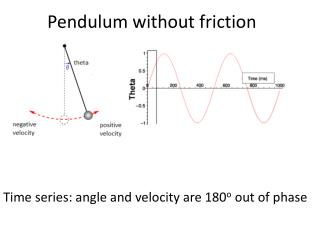DownloadDownload PresentationPendulum without friction

# Pendulum without friction

Download Presentation## Pendulum without friction

- - - - - - - - - - - - - - - - - - - - - - - - - - - E N D - - - - - - - - - - - - - - - - - - - - - - - - - - -

1. ### Pendulum without friction

Time series: angle and velocity are 180o out of phase
2. ### Pendulum without friction

http://webphysics.davidson.edu/applets/animator4/demo_pendulum.html Phase space: plot angle vs velocity. This shows a “limit cycle” in phase space.
3. ### Pendulum without friction

Larger initial angles put you in a larger limit cycle.
4. ### Pendulum with friction: fixed point

http://www.mcasco.com/Order/oap2dpsd.html “Fixed point” attractor in phase space: gradually spirals in to one point.
5. ### Pendulum with friction: basin of attraction

Different starting positions end up in the same fixed point. Its like rolling a marble into a basin. No matter where you start from, it ends up in the drain.
6. ### Pendulum with friction

Adding a third dimension of potential energy: the basin of attraction as a gravitational well.
7. ### Inverted Pendulum: ball on flexible rod flops to one side or the other

Basin of attraction in phase space: two fixed points.
8. ### Inverted Pendulum: ball on flexible rod

Potential energy plot shows the two fixed points as the “landscape” of the basin of attraction.
9. ### Bi-polar emotions as phase space

If someone flips between “manic” and “depressive” moods, we can model as two basins of attraction. Fixed point attractors would eventually settle.
10. ### Driven Inverted Pendulum

Move the inverted pendulum back and forth: At first period 2, then period 4, then… chaos! Chaotic attractor impossible to predict in the long run because of sensitivity to initial conditions
11. ### Emotional phase space

Imagine a wolf captured for a zoo: it might cycle between fear and aggression unpredictably.
12. Behavioral “modes” for fish schools

### A. Swarm: low P, low MB. Torus: low P, high MC. Parallel: high P, low M

P = Group polarization -- how much of group is aligned M = Group angular momentum -- how much of group rotates about center
13. Fish school behavior: basins of attraction Swarm: low P, low MTorus: low P, high MParallel: high P, low M P = Group polarization -- how much of group is aligned M = Group angular momentum -- how much of group rotates about center
14. Human school behavior: basins of attraction Phase space of cliques (roles, stereotypes) as basins of attraction for high school
15. Network behavior and basins of attraction Stuart Kaufman modeled genetic networks as Boolean nodes (1 or 0). N nodes with K (max) inputs and one output. At every time-step, each node changes its state (“lights up”) depending on its internal rules. Since there are 2n possible states, we might assume it just cycles through some huge number before it repeats
16. Network behavior and basins of attraction But in fact it quickly settles into just a few basins of attraction http://www-users.cs.york.ac.uk/susan/cyc/n/nk.htm.
17. Network behavior and basins of attraction Kauffman speculates that each basin of attraction could roughly correspond to a different cell type. His model predicts a power law for how number of cell types rises with number of genes. The actual rise in number of cell types with number of genes is not far off (although this is controversial).
18. Social networks and basins of attraction Hubs are one way to think about basins of attraction in social networks: highly connected nodes are “attractive” (recall the theory of “preferential attachment” to explain scale-free degree distribution). But web links are relatively slow to change -- a more dynamic model would ask about basins of information flow (think of photo memes)